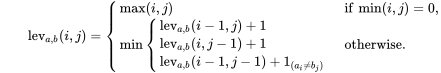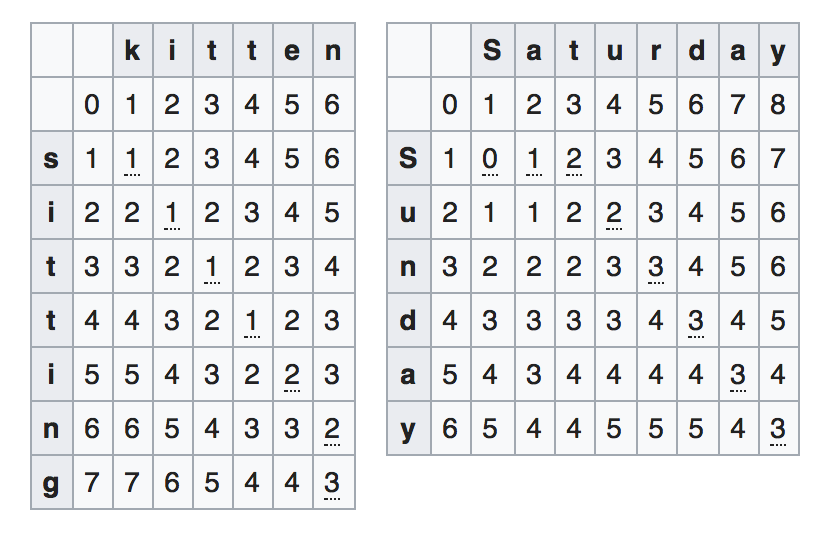# Swift – Calculate Levenshtein distance

Ciao,

here a conversion on an old tutorial in Swift about the Levenshtein distance of two strings.In information theorylinguistics and computer science, the Levenshtein distance is a string metric for measuring the difference between two sequences. Informally, the Levenshtein distance between two words is the minimum number of single-character edits (insertions, deletions or substitutions) required to change one word into the other. It is named after the Soviet mathematician Vladimir Levenshtein, who considered this distance in 1965.

Levenshtein distance may also be referred to as edit distance, although that term may also denote a larger family of distance metrics.:32 It is closely related to pairwise string alignments.

I’ve implemented the “Iterative full matrix” algorithm.First of all create a Array2d class:

```class Array2D {
var cols:Int, rows:Int
var matrix: [Int]

init(cols:Int, rows:Int) {
self.cols = cols
self.rows = rows
matrix = Array(repeating: 0, count: cols * rows)
}

subscript(col:Int, row:Int) -> Int {
get { return matrix[cols * row + col] }
set { matrix[cols*row+col] = newValue }
}

func colCount() -> Int { return self.cols }
func rowCount() -> Int { return self.rows }
}```

Next thing is a custom min function used to reduce the values:

```func min(_ numbers: Int...) -> Int {
return numbers.reduce(numbers, {\$0 < \$1 ? \$0 : \$1})
}```

and last one the core function to get your distance:

```class func levenshteinDistance(aStr: String, bStr: String) -> Int {
let a = Array(aStr.utf16)
let b = Array(bStr.utf16)

if a.count == 0 || b.count == 0 { return 100 }

let dist = Array2D(cols: a.count + 1, rows: b.count + 1)

for i in 1...a.count {
dist[i, 0] = i
}

for j in 1...b.count {
dist[0, j] = j
}

for i in 1...a.count {
for j in 1...b.count {
if a[i-1] == b[j-1] {
dist[i, j] = dist[i-1, j-1]  // noop
} else {
dist[i, j] = StringUtils.min(
dist[i-1, j  ] + 1,  // deletion
dist[i,   j-1] + 1,  // insertion
dist[i-1, j-1] + 1   // substitution
)
}
}
}

return dist[a.count, b.count]
}```

Now you can use in your projects!

```if levenshteinDistance(aStr: "milano", bStr: "milao") < 3 {
// do something
}```